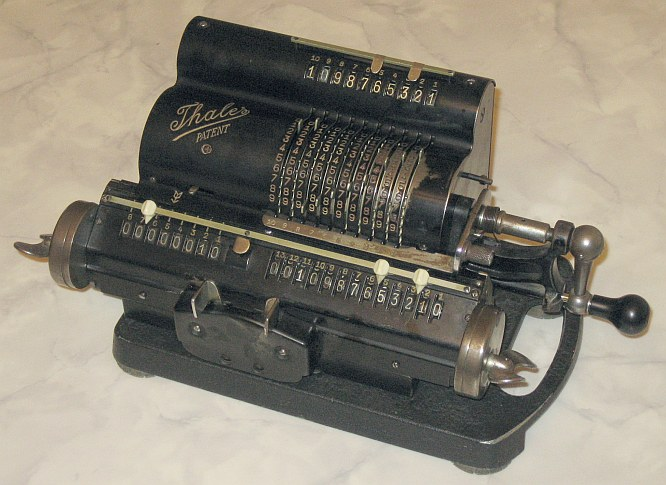previous <<==>> next

 THALES - CE2 DecimalMarkers on top were missing - Now with "home-made" ones PROSPECT from 1938 ... Have a look at the  THALES  GEO ... Have a look at the THALES »KA« ADDING MACHINES ...
 T E C H N I C A L D A T A of the Mechanical Calculator THALES »CE« *************************************************************************** Serial Number: 59982 Dimensions: (ca.) Width = 11_3/8" / 29 cm Depth = 6_1/4" / 16 cm Height = 5_1/2" / 14 cm Weight: (ca.) 12_3/4 lbs / 5.8 kg Mechanics: Pin-Wheel / Sprossenrad 10s Carry Mechanism Functions: Add, Subtract, Multiply, Divide Registers: Input = 10 Decimals Counter = 8 Decimals Arithmetic = 13 Decimals Manufacturer: THALES-WERK Rastatt / Baden Germany 1938 H O W T O U S E the THALES CE ************************************* BASIC SETTINGS: =============== (a) Crank: ---------- For addition (+) the crank is turned clockwise; for subtraction (-) the crank is turned counter-clockwise. In the idle position the crank is DOWN & LOCKED. To make one or more turns with the crank, pull out the handle to unlock. When finished the turn(s), let the handle snap into lock again. The locked down position only makes other functions (ex. clearing) accessible. REMARK: Every started turn has to be finished completely! Accidentally started turns are correctible somehow. (b) Clearing Registers: ----------------------- With the BIG LEVER on top the INPUT can be cleared. (It is intended to use the thumb for). The LEFT WING-NUT clears the COUNTER; the RIGHT WING-NUT clears the COUNTER & ARITHMETIC - Exception: When the KEY in the middle is pressed simultaniously, the ARITHMETIC ONLY is cleared. (c) Shifting the Carriage: -------------------------- With the 2-grip lever the carriage can be moved one step right or one step left - or, when pushed together, the carriage can be shifted free. The left position "1" is the "Start Position" of the carriage. (d) Counting Direction: ----------------------- It depends of the 1st turn of the crank how the counter is switched to (+) or (-). The counter will increase its content for positive (clock- wise) or negative (counter-clockwise) turns. When the direction of the turns is changed, the counter will decrease its content. In both cases the counter is working with 10s-carry - and allows "Shortened Method of Multiplication". ADDITION & SUBTRACTION: ======================= Example: 123 + 45 - 6 = 162 Clear input, counter and arithmetic units. ADD: Enter the first number (123) in the far right of the input unit. Make a positive (clockwise) turn with the crank to transfer the number into arithmetic unit. The counting unit displays the figure 1. Enter the second number (45). Make a positive (clockwise) turn with the crank to add the number. The arithmetic unit displays the intermediate sum (168) and the counting unit displays the figure 2. SUBTRACT: Enter the third number (6). Make a negative (counter-clockwise) turn with the crank. The arithmetic unit displays the result (162) and the counting unit is decreased by 1. REMARK: NEGATIVE RESULTS are displayed in the arithmetic unit as the COMPLEMENT of the next higher 10, 100, 1000, ... Example: -12 = 99...9988 MULTIPLICATION: =============== Example: 123 x 45 = 5535 Clear input, counter and arithmetic units. Enter the multiplicand (123) in the far right of the input unit. The multiplicator (45) has two digits, so the carriage is shifted to position 2. Make positive (clockwise) turns with the crank, until the first figure of the multiplicator (4) will appear in the 2nd position of the counter unit. Shift the carriage to position 1. Repeat making positive turns with the crank, until the second figure of the multiplicator (5) appears in the 1st position of the counter unit. The multiplication is done: The multiplicand (123) stays in the input unit, the multiplicator (45) in the counter and the result (5535) is in the arithmetic unit. DIVISION: ========= Example: 22 : 7 = 3.1428571 Remainder 3 Division requires 3 steps: (A) To Set the Divisor into Input Unit: --------------------------------------- Enter the DIVISOR (7) in the far right of the input unit. (B) To Set the Dividend into Arithmetic Unit: --------------------------------------------- For the maximum number of decimals, pull out the carriage to the far right. Set the DIVIDENT (22) into the arithmetic unit with the affiliated toothed wheels appropriately under the divisor. (C) To Divide: -------------- Set the counting direction to (-). Make negative (counter-clockwise) turns with the crank until the arithmetic unit shows an "underflow". Make one positive (clockwise) turn with the crank. Move the carriage to the next left position. Repeat this procedure until the required number of decimals ... The result (3.1428571) is in the counter unit, and the remainder (3) is in the arithmetic unit. The divisor (7) stays in the input unit, therefore an additional decimal can be estimated... ( 4, cause 4 x 7 = 28). impressum: *************************************************************************** © C.HAMANN http://public.BHT-Berlin.de/hamann 11/01/21